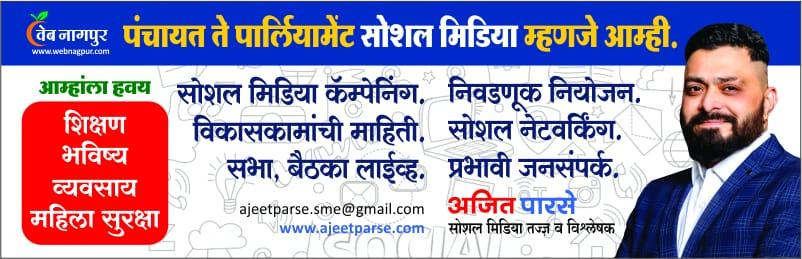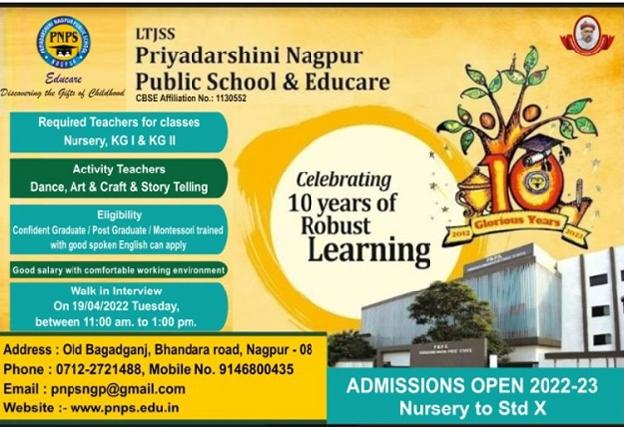Published On : Tue, Aug 10th, 2021
Featured | By Nagpur Today

# Strategies to Score More than 90% Marks in Class 7 Maths ExamStudents can easily score more than 90% marks in the Class 7 Maths exam. They need to practice it daily. There are a total of 17 chapters in Class 7 Maths. Students can understand each topic by listening to the teacher properly in the class and practising maths every day at home. Students should solve all the questions given in NCERT class 7 maths. NCERT book is enough to practice class 7 maths. They can also refer to extra books such as R D Sharma class 7 solutions and R S Aggarwal Class solutions as reference books.

Maths can be an interesting subject, if done the right way. Practice is the key to securing good marks in maths. Students should dedicate at least an hour every day to solving math problems.

The main topics and strategies that can help you score more than 90% in the class 7 maths exam are –

1. In class 7, the first chapter is about Integers. Students will learn about the properties of integers. After studying whole numbers in the 6th class, students will learn the complete concept of integers in the first chapter. They will learn about addition, subtraction, multiplication, and division of integers.
2. The second chapter covers Fractions and Decimals. Students have learnt about fractions and decimals in class 6 as well. This is a further exploration of the topic. In class 7, students will learn about the different operations on fractions and decimals such as addition, subtraction, multiplication, and division. They will also learn how to represent them on the number line.
3. The next chapter is about Data Handling. This is an interesting and easy chapter as it covers the topics of data accumulation, interpretation, and plotting of graphs from the given data. It deals with real-life situations and students enjoy solving such questions. They also learn about different terms such as mean, median, mode, and range. Students will also learn about probability in this chapter.
4. Chapter four is about Simple Equations. It involves forming a simple equation after reading the question and solving it. It also includes problem-solving questions.
5. In chapter five, students learn about Lines and Angles. This chapter includes the properties of parallel lines and different angles formed by two parallel lines when a transversal line crosses over them. Students will learn about alternate angles, vertical angles, complementary angles, supplementary angles, adjacent angles, etc.
6. Chapter 6 is about The Triangle And Its Properties. The chapter helps students to learn about the different triangles, the properties of triangles, and also the Pythagoras theorem. They will learn about the different applications of this theorem through word problems.
7. The next chapter is about the Congruence of Triangles. It is the specific property of triangles and in this chapter, students will learn about this property through different problems. The property of congruence is discussed in detail for a comprehensive understanding of the concept.
8. Chapter eight is about Comparing Quantities. It is an interesting chapter as it helps students to learn the techniques for measurement and comparing quantities. The tools used for comparing quantities include ratio, percentage, profit and loss, as well as how to calculate interest. Students learn about different concepts through solving problems and calculations that are related to real-life situations.
9. Chapter nine is about Rational Numbers. In this chapter, students will learn about rational numbers in detail. They will learn about the properties of rational numbers.
10. Chapter 10 is very easy and it is about Practical Geometry. Students will learn about how to construct lines and angles using geometrical tools. Students have to follow the same basic steps for constructing different lines and angles.
11. Chapter 11 in class 7 math deals with the Perimeter and Area of the common shapes. It is the continuation of the mensuration concept taught in class 6. It is a simple chapter and children will solve new problems related to simple shapes. Complex shapes are not introduced in class 7. Students will be solving a wide range of word problems related to finding the area and perimeter of common shapes such as square, rectangle, and circle.
12. Chapter 12 is about Algebraic Equations. It is also an interesting chapter and students enjoy learning it. They have to form algebraic equations from simple word problems and solve them. Word problems are related to real-life situations where students can use algebra for solving problems. Students will also learn about basic rules for forming an algebraic expression.
13. Chapter 13 is about Exponents and Powers. It is a new concept. Students find it very interesting as they will study the different properties of exponents in this chapter. They will also learn how to express very large numbers into the standard form which is also called scientific notation.
14. Chapter 14 is very easy and students do not have to do long calculations in this chapter. Chapter 14 is about the Symmetry of Different Shapes. Symmetry is important in life as it helps craftsmen to make different designs and patterns. In this chapter, students learn the basic concepts of symmetry and its importance in life. It is a very short chapter.
15. The last chapter is about Visualizing Solid Shapes. It is a new concept for students and deals with the introduction and basic properties of solid shapes such as cubes, cuboids, cones, cylinders, prisms, etc. Students will learn about the features of different solid shapes and how to use them in real life.

The most important thing for scoring high marks in maths is practising regularly. Consistent practice helps students remember the concepts throughout life.  Students should solve all the exercise questions given in the NCERT book.

Practical application of maths concepts in real life is one of the best ways for students to learn and understand the concepts. Mugging the formulas will not help them solve problems quickly. They should focus on examples given in every chapter in the NCERT book. Before solving the exercise questions, they should also solve examples. This will help them to understand the concept properly and score more than 90 in their maths exam.